• 简而言之，链表
• 简而言之地图
• 编码挑战

# Linked lists in a nutshell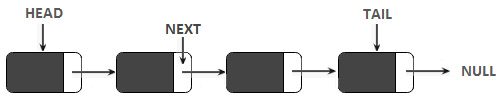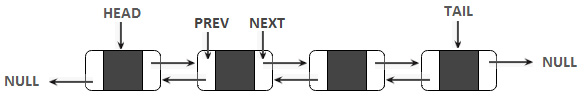```private final class Node {
private int data;
private Node next;
}```

```private final class Node {
private int data;
private Node next;
private Node prev;
}```

# Maps in a nutshell

• 键是唯一的（即不允许重复键）。
• 我们可以查看键列表、值列表或两者。
• 使用地图最常用的方法是 `get()``put()````删除（） 。```

# Coding challenges

• 创建不可修改/不可变的集合
• 映射默认值
• 计算 `Map` 中是否存在/不存在值
• `Map` 中移除
• 替换 `Map` 中的条目
• 比较两张地图
• `Map` 进行排序
• 复制 `HashMap`
• 合并两张地图
• 删除集合中与谓词匹配的所有元素

## Coding challenge 1 – Map put, get, and remove

```private final class MyEntry<K, V> {
private final K key;
private V value;
public MyEntry(K key, V value) {
this.key = key;
this.value = value;
}
// getters and setters omitted for brevity
}```

```private static final int DEFAULT_CAPACITY = 16;
private MyEntry<K, V>[] entries
= new MyEntry[DEFAULT_CAPACITY];```

```private int size;
public void put(K key, V value) {
boolean success = true;
for (int i = 0; i < size; i++) {
if (entries[i].getKey().equals(key)) {
entries[i].setValue(value);
success = false;
}
}
if (success) {
checkCapacity();
entries[size++] = new MyEntry<>(key, value);
}
}```

```private void checkCapacity() {
if (size == entries.length) {
int newSize = entries.length * 2;
entries = Arrays.copyOf(entries, newSize);
}
}```

```public V get(K key) {
for (int i = 0; i < size; i++) {
if (entries[i] != null) {
if (entries[i].getKey().equals(key)) {
return entries[i].getValue();
}
}
}
return null;
}```

```public void remove(K key) {
for (int i = 0; i < size; i++) {
if (entries[i].getKey().equals(key)) {
entries[i] = null;
size--;
condenseArray(i);
}
}
}
private void condenseArray(int start) {
int i;
for (i = start; i < size; i++) {
entries[i] = entries[i + 1];
}
entries[i] = null; // don't forget this line
}```

## Coding challenge 2 – Map the key set and values

```public Set<K> keySet() {
Set<K> set = new HashSet<>();
for (int i = 0; i < size; i++) {
}
return set;
}```

```public Collection<V> values() {
List<V> list = new ArrayList<>();
for (int i = 0; i < size; i++) {
}
return list;
}```

## Coding challenge 3 – Nuts and bolts

```char[] nuts = {'\$', '%', '&', 'x', '@'};
char[] bolts = {'%', '@', 'x', '\$', '&'};```

```public static void match(char[] nuts, char[] bolts) {
// in this map, each nut is a key and
// its position is as value
Map<Character, Integer> map = new HashMap<>();
for (int i = 0; i < nuts.length; i++) {
map.put(nuts[i], i);
}
//for each bolt, search a nut
for (int i = 0; i < bolts.length; i++) {
char bolt = bolts[i];
if (map.containsKey(bolt)) {
nuts[i] = bolts[i];
} else {
System.out.println("Bolt " + bolt + " has no nut");
}
}
System.out.println("Matches between nuts and bolts: ");
System.out.println("Nuts: " + Arrays.toString(nuts));
System.out.println("Bolts: " +Arrays.toString(bolts));
}```

## Coding challenge 4 – Remove duplicates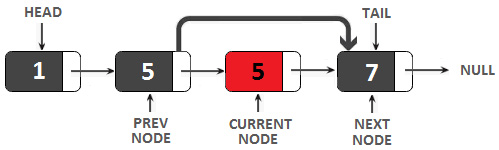```// 'size' is the linked list size
public void removeDuplicates() {
Set<Integer> dataSet = new HashSet<>();
Node prevNode = null;
while (currentNode != null) {
if (dataSet.contains(currentNode.data)) {
prevNode.next = currentNode.next;
if (currentNode == tail) {
tail = prevNode;
}
size--;
} else {
prevNode = currentNode;
}
currentNode = currentNode.next;
}
}```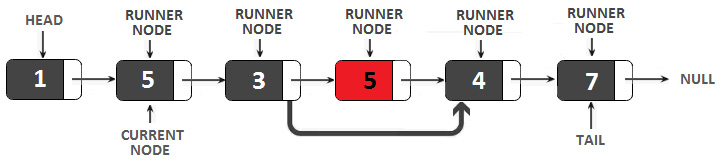1. 当前节点，从链表的头部开始，逐个节点遍历链表，直到到达尾部（例如上图中，当前节点为第二个节点）。
2. runner节点，从与当前节点相同的地方开始，即链表的头部。

```public void removeDuplicates() {
while (currentNode != null) {
Node runnerNode = currentNode;
while (runnerNode.next != null) {
if (runnerNode.next.data == currentNode.data) {
if (runnerNode.next == tail) {
tail = runnerNode;
}
runnerNode.next = runnerNode.next.next;
size--;
} else {
runnerNode = runnerNode.next;
}
}
currentNode = currentNode.next;
}
}```

## Coding challenge 5 – Rearranging linked lists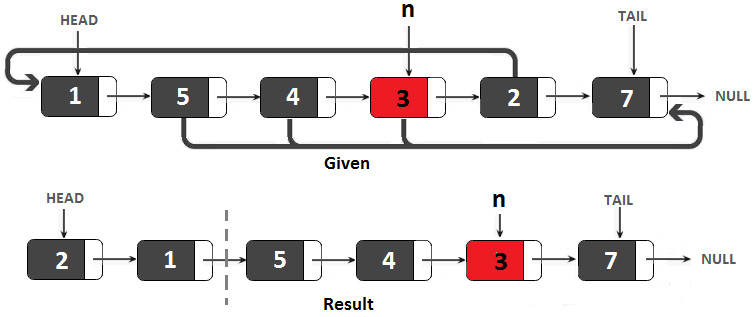```public void rearrange(int n) {
tail = currentNode;
while (currentNode != null) {
Node nextNode = currentNode.next;
if (currentNode.data < n) {
// insert node at the head
} else {
// insert node at the tail
tail.next = currentNode;
tail = currentNode;
}
currentNode = nextNode;
}
tail.next = null;
}```

## Coding challenge 6 – The nth to last node

Solution: We have a bunch of nodes and we have to find the nth node that satisfies a given constraint. Based on our experience from Chapter 8, Recursion and Dynamic Programming, we can intuit that this problem has a solution involving recursion. But we can also solve it via an iterative solution. Since the iterative solution is more interesting, I will present it here, while the recursive solution is available in the bundled code.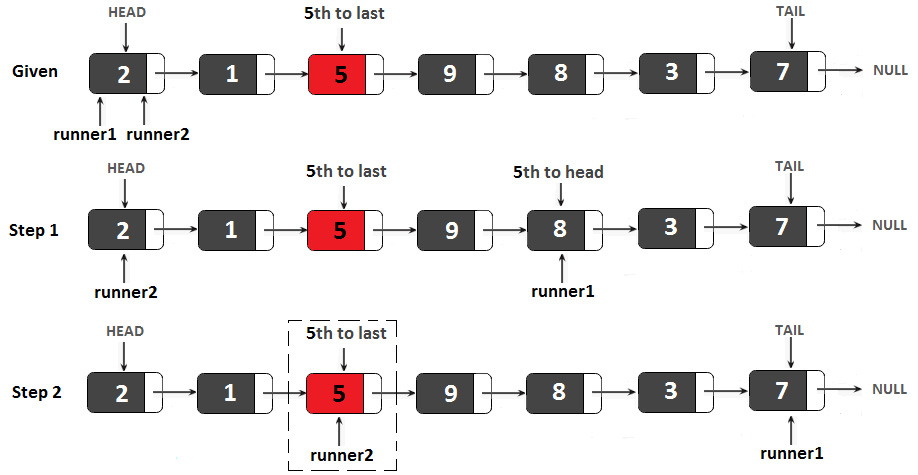```public int nthToLastIterative(int n) {
// both runners are set to the start
// runner1 goes in the nth position
for (int i = 0; i < n; i++) {
if (firstRunner == null) {
throw new IllegalArgumentException(
"The given n index is out of bounds");
}
firstRunner = firstRunner.next;
}
// runner2 run as long as runner1 is not null
// basically, when runner1 cannot run further (is null),
// runner2 will be placed on the nth to last node
while (firstRunner != null) {
firstRunner = firstRunner.next;
secondRunner = secondRunner.next;
}
return secondRunner.data;
}```

## Coding challenge 7 – Loop start detection

### The Fast Runner/Slow Runner approach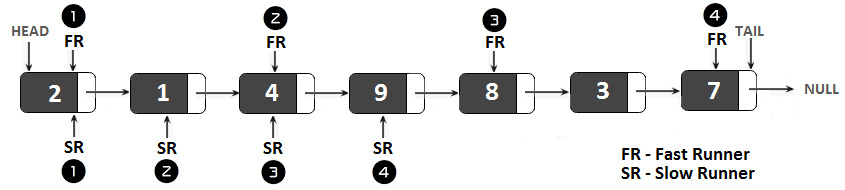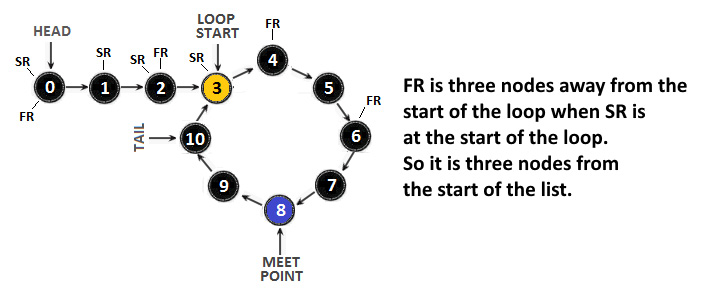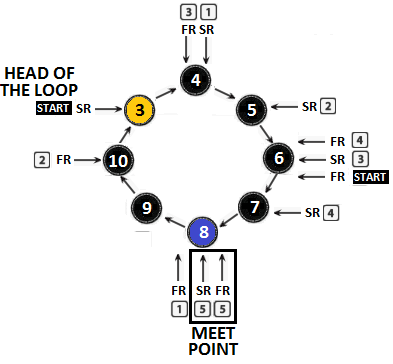1. 从链表头部的 FRSR 开始。
2. 以 1 个节点的速率移动 SR，以 2 个节点的速率移动 FR
3. 当它们发生碰撞时（在交汇点），将 SR 移动到链表的头部，并将 FR 保持在原处。
4. 以 1 个节点的速度移动 SRFR 直到它们发生碰撞（这是表示 循环）。

```public void findLoopStartNode() {
// fastRunner meets slowRunner
while (fastRunner != null && fastRunner.next != null) {
slowRunner = slowRunner.next;
fastRunner = fastRunner.next.next;
if (slowRunner == fastRunner) { // they met
System.out.println("\nThe meet point is at
the node with value: " + slowRunner);
break;
}
}
// if no meeting point was found then there is no loop
if (fastRunner == null || fastRunner.next == null) {
return;
}
// the fastRunner remains at the meeting point
// they move simultaneously node-by-node and
// they should meet at the loop start
while (slowRunner != fastRunner) {
slowRunner = slowRunner.next;
fastRunner = fastRunner.next;
}
// both pointers points to the start of the loop
System.out.println("\nLoop start detected at
the node with value: " + fastRunner);
}```

## Coding challenge 8 – Palindromes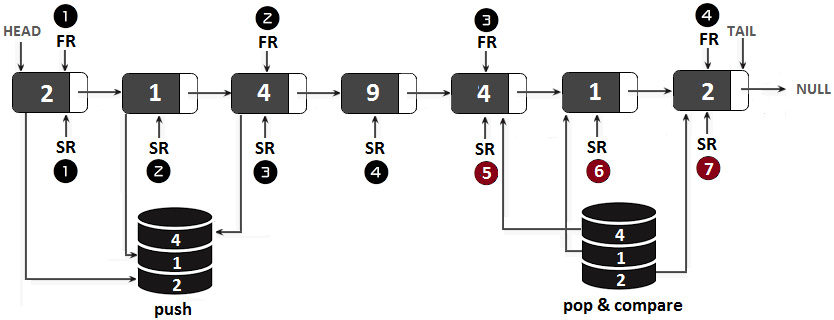```public boolean isPalindrome() {
Stack<Integer> firstHalf = new Stack<>();
// the first half of the linked list is added into the stack
while (fastRunner != null && fastRunner.next != null) {
firstHalf.push(slowRunner.data);
slowRunner = slowRunner.next;
fastRunner = fastRunner.next.next;
}
// for odd number of elements we to skip the middle node
if (fastRunner != null) {
slowRunner = slowRunner.next;
}
// pop from the stack and compare with the node by node of
// the second half of the linked list
while (slowRunner != null) {
int top = firstHalf.pop();
// a mismatch means that the list is not a palindrome
if (top != slowRunner.data) {
return false;
}
slowRunner = slowRunner.next;
}
return true;
}```

## Coding challenge 9 – Sum two linked lists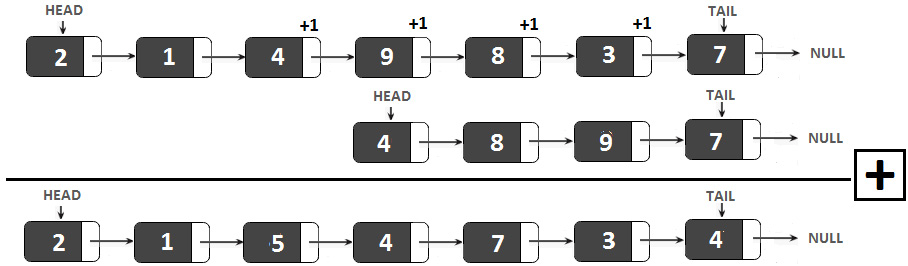```private Node sum(Node node1, Node node2, int carry) {
if (node1 == null && node2 == null && carry == 0) {
return null;
}
Node resultNode = new Node();
int value = carry;
if (node1 != null) {
value += node1.data;
}
if (node2 != null) {
value += node2.data;
}
resultNode.data = value % 10;
if (node1 != null || node2 != null) {
Node more = sum(node1 == null
? null : node1.next, node2 == null
? null : node2.next, value >= 10 ? 1 : 0);
resultNode.next = more;
}
return resultNode;
}```

## Coding challenge 10 – Linked lists intersection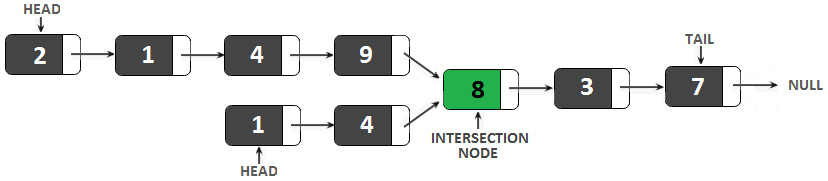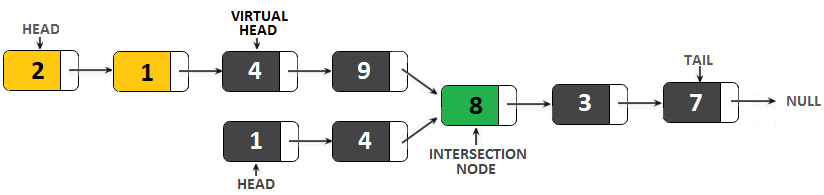1. 确定列表的大小。
2. 如果第一个列表（我们将其表示为 l1）比第二个列表（我们将其表示为 l2）长，那么将第一个列表的指针移动到 (l1-l2)。
3. 如果第一个列表比第二个短，则将第二个列表的指针移动到 (l2-l1)。
4. 逐个节点移动两个 指针，直到到达终点或它们发生碰撞。

```public int intersection() {
// this is the head of first list
// this is the head of the second list
// compute the size of both linked lists
// linkedListSize() is just a helper method
// the first linked list is longer than the second one
if (s1 > s2) {
for (int i = 0; i < (s1 - s2); i++) {
currentNode1 = currentNode1.next;
}
} else {
// the second linked list is longer than the first one
for (int i = 0; i < (s2 - s1); i++) {
currentNode2 = currentNode2.next;
}
}
// iterate both lists until the end or the intersection node
while (currentNode1 != null && currentNode2 != null) {
// we compare references not values!
if (currentNode1 == currentNode2) {
return currentNode1.data;
}
currentNode1 = currentNode1.next;
currentNode2 = currentNode2.next;
}
return -1;
}```

## Coding challenge 11 – Swap adjacent nodes

Solution：我们可以减少寻找交换两个连续节点n1n2。交换两个值（例如，两个整数，v1v2）的著名技术依赖于辅助变量和可以写成如下：

aux = v1; v1 = v2； v2 = 辅助；

aux = n1; n1 = n2; n2 = 辅助；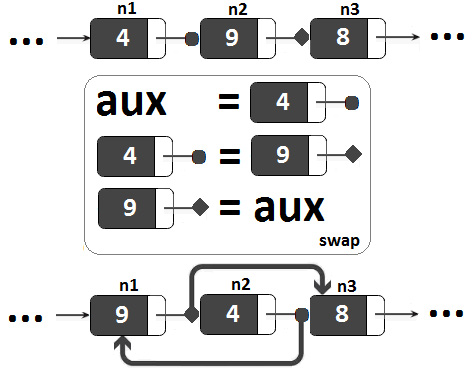n1.next = n2

n2.next = n3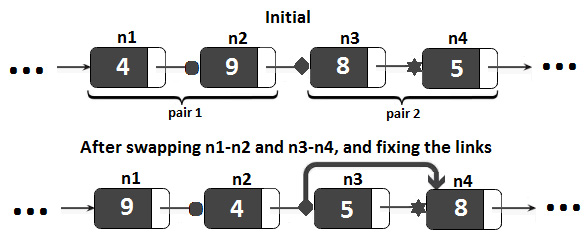```public void swap() {
return;
}
Node prevPair = null;
// consider two nodes at a time and swap their links
while (currentNode != null && currentNode.next != null) {
Node node1 = currentNode;           // first node
Node node2 = currentNode.next;      // second node
Node node3 = currentNode.next.next; // third node
// swap node1 node2
Node auxNode = node1;
node1 = node2;
node2 = auxNode;
// repair the links broken by swapping
node1.next = node2;
node2.next = node3;
// if we are at the first swap we set the head
if (prevPair == null) {
} else {
// we link the previous pair to this pair
prevPair.next = node1;
}
// there are no more nodes, therefore set the tail
if (currentNode.next == null) {
tail = currentNode;
}
// prepare the prevNode of the current pair
prevPair = node2;
// advance to the next pair
currentNode = node3;
}
}```

## Coding challenge 12 – Merge two sorted linked lists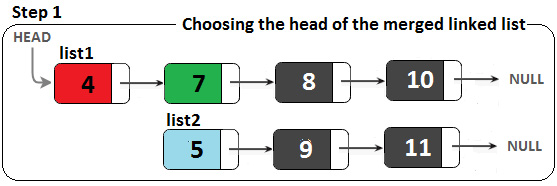```Node auxNode = list1.next; // auxNode = node with value 7
list1.next = list2;        // list1.next = node with value 5
list2 = auxNode;           // list2 = node with value 7```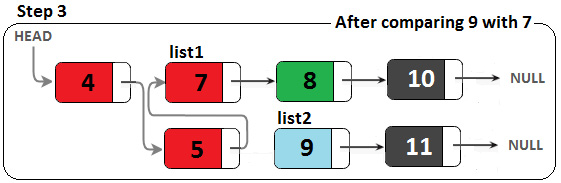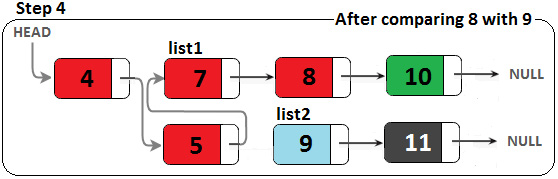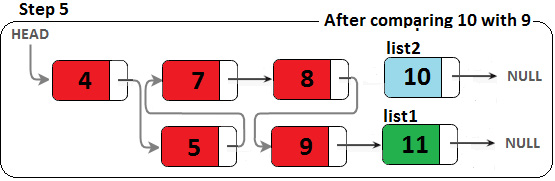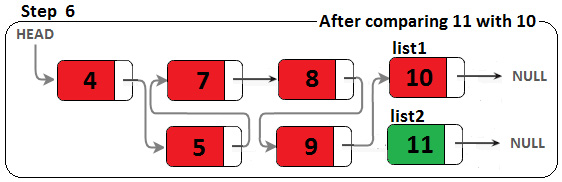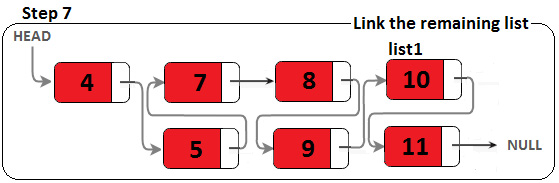```public void merge(SinglyLinkedList sll) {
// these are the two lists
Node list2 = sll.head;  // from this list we add nodes at
// appropriate place in list1
// compare heads and swap them if it is necessary
if (list1.data < list2.data) {
} else {
list2 = list1;
}
// compare the nodes from list1 with the nodes from list2
while (list1.next != null) {
if (list1.next.data > list2.data) {
Node auxNode = list1.next;
list1.next = list2;
list2 = auxNode;
}
list1 = list1.next;
}
if (list1.next == null) {
list1.next = list2;
}
}```

## Coding challenge 13 – Remove the redundant path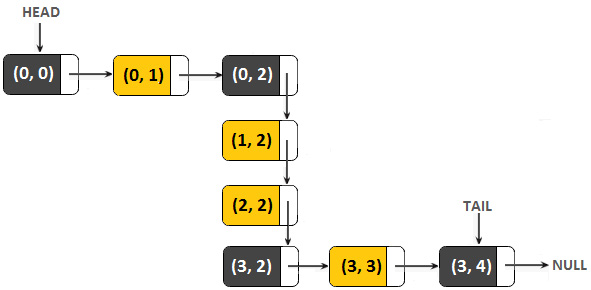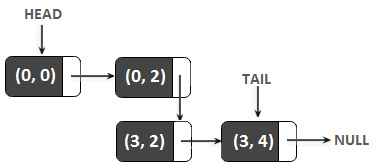```public void removeRedundantPath() {
while (currentNode.next != null
&& currentNode.next.next != null) {
Node middleNode = currentNode.next.next;
// check for a vertical triplet (triplet with same column)
if (currentNode.c == currentNode.next.c
&& currentNode.c == middleNode.c) {
// delete the middle node
currentNode.next = middleNode;
} // check for a horizontal triplet
else if (currentNode.r == currentNode.next.r
&& currentNode.r == middleNode.r) {
// delete the middle node
currentNode.next = middleNode;
} else {
currentNode = currentNode.next;
}
}
}```

## Coding challenge 14 – Move the last node to the front

1. 将指针移动到倒数第二个节点（我们将其表示为 currentNode）。
2. 存储 currentNode.next（我们将其表示为 nextNode - 这是最后一个节点）。
3. `cu`rrentNode.next 设置为 `null` （因此，最后一个节点变为尾巴）。

```public void moveLastToFront() {
// step 1
while (currentNode.next.next != null) {
currentNode = currentNode.next;
}
// step 2
Node nextNode = currentNode.next;
// step 3
currentNode.next = null;
// step 4
}```

1. 将指针移动到倒数第二个节点（我们将其表示为 currentNode）。
2. 将链表 转换为循环链表（将 currentNode.next.next 链接到头部）。
3. 将新头设置为 currentNode.next
4. 通过将 currentNode.next 设置为 `null` 来打破循环。

```public void moveLastToFront() {
// step 1
while (currentNode.next.next != null) {
currentNode = currentNode.next;
}
// step 2
// step 3
// step 4
currentNode.next = null;
}```

## Coding challenge 15 – Reverse a singly linked list in groups of k

1. 从 0 开始的计数器。
2. As the current node (initially the head) is not `null` and we haven't reached the given k, the following occurs:

一个。 next 节点（最初是 `null`）成为 current 旁边的节点节点（最初是头部）。

湾。 current 节点旁边的节点（最初是 head）成为 previous 节点（最初是 `空`）。

C。 previous 节点成为 current 节点（最初是头部）。

d。 当前节点成为下一个节点（来自step 2a的节点） .

e.增加计数器。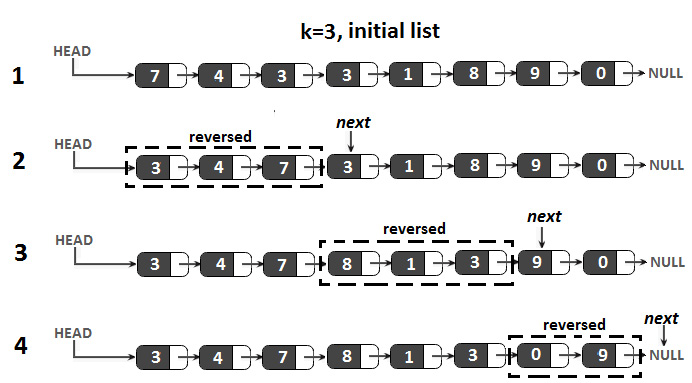```public void reverseInKGroups(int k) {
}
}
private Node reverseInKGroups(Node head, int k) {
Node next = null;
Node prev = null;
int counter = 0;
// reverse first 'k' nodes of linked list
while (current != null && counter < k) {
next = current.next;
current.next = prev;
prev = current;
current = next;
counter++;
}
// 'next' points to (k+1)th node
if (next != null) {
}
// 'prev' is now the head of the input list
return prev;
}```

## Coding challenge 16 – Reverse a doubly linked list

```public void reverse() {
Node prevNode = null;
while (currentNode != null) {
// swap next and prev pointers of the current node
Node prev = currentNode.prev;
currentNode.prev = currentNode.next;
currentNode.next = prev;
// update the previous node before moving to the next node
prevNode = currentNode;
// move to the next node in the doubly linked list
currentNode = currentNode.prev;
}
// update the head to point to the last node
if (prevNode != null) {
}
}```

## Coding challenge 17 – LRU cache

• 固定大小：缓存必须使用有限的内存量。因此，它需要有一些界限（例如，固定大小）。
• 快速访问数据：插入和搜索操作应该很快；最好是 O(1) 复杂度时间。
• 快速驱逐数据：当缓存已满时（已达到分配的界限），缓存应该授权一种高效的算法来驱逐条目。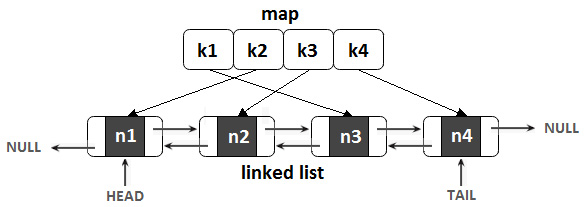• 在缓存中插入一个新的 条目将导致将相应的节点添加到链表的头部（因此，链表的头部保存了最近使用的值）。
• 当一个条目被访问时，我们将其对应的节点移动到链表的头部。
• 当我们需要驱逐一个条目时，我们驱逐链表的尾部（因此，链表的尾部保存了最近最少使用的值）。

```public final class LRUCache {
private final class Node {
private int key;
private int value;
private Node next;
private Node prev;
}
private final Map<Integer, Node> hashmap;
private Node tail;
// 5 is the maximum size of the cache
private static final int LRU_SIZE = 5;
public LRUCache() {
hashmap = new HashMap<>();
}
public int getEntry(int key) {
Node node = hashmap.get(key);
// if the key already exist then update its usage in cache
if (node != null) {
removeNode(node);
return node.value;
}
return -1;
}
public void putEntry(int key, int value) {
Node node = hashmap.get(key);
// if the key already exist then update
// the value and move it to top of the cache
if (node != null) {
node.value = value;
removeNode(node);
} else {
// this is new key
Node newNode = new Node();
newNode.prev = null;
newNode.next = null;
newNode.value = value;
newNode.key = key;
// if we reached the maximum size of the cache then
// we have to remove the  Least Recently Used
if (hashmap.size() >= LRU_SIZE) {
hashmap.remove(tail.key);
removeNode(tail);
} else {
}
hashmap.put(key, newNode);
}
}
// helper method to add a node to the top of the cache
node.prev = null;
}
if (tail == null) {
}
}
// helper method to remove a node from the cache
private void removeNode(Node node) {
if (node.prev != null) {
node.prev.next = node.next;
} else {
}
if (node.next != null) {
node.next.prev = node.prev;
} else {
tail = node.prev;
}
}
}```